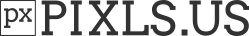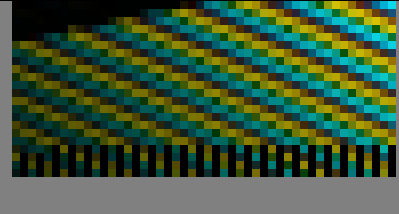# G'MIC math evaluator now deals with vectors (and matrices, and custom functions!)

Just wanted to again tell you folks I’m stoked with this new ability. Thanks again Souphead and David for making this happen.I been reading up on this, but how I insert new value on a matrix in a loop, and the matrix resize after addition? I’m looking into a dynamic matrix or something like that.

``````m={w-1}

n={h-1}

k=0

l=0

do

k+=1

while \$k<=\$m&&\$l<=\$n
``````

I’m working on replicating this code here (You seen it before on the glitch art again thread) - Effective Programming: spiral printing of a two dimensional array

My goal is to copy that code, and then use fill " i(matrix(x+y)%w,floor(matrix(x+y)/w) "

Also, I know that in C++, you could add values by v.pushback(). So, I believe this has to do with vector.

I still avoid vectors and matrices and C / C++.I think recent versions allow unspecified vector sizes, so adding an element or set of elements should be simpler than C++'s pushes, pops, etc.

How so? I have not really found amything about unspecified vector in gmic and push equilavent.

Should be plenty of examples in the stdlib or exercises thread. Basically, declare a vector, make a for-loop and then append values to each element. Since the vector doesn’t require a predefined size anymore, you may add as many values as you desire. That is the gist of it.

What I feel more comfortable doing is making an image of values, which is essentially a matrix. The great thing about that is that this matrix is portable outside of the fill command and you could easily apply scalar, vector and element operations on it using builtin commands, which means that you could break up complex operations that you are known for into smaller chunks.

From looking at the stdlib.

Ve = int() is basically a vector. But, I haven’t really found anything about appending a value in vector in the g’mic reference, and stdlib only helped with finding what’s a vector. append or ‘a’ command is the closest i can think of.

EDIT: I found poisson disk though it’s a bit confusing to figure out. But, I doubt fill will answer my problem as the original code output multiple time per “fill”, so that’s going to be a issue. fill is basically just one output in one channel at the minimum. Something like that.

Also, I found this code here - G'MIC exercises - #187 by David_Tschumperle

I must look at this more because this is all very new to me.

The vector-valued variables in the math parser have a fixed size, determined at the compile-time when the variable is defined.
To manage vectors with a dynamic size, there is anyway the solution to define an image (e.g a 1x1 image), and use the functions `dar_insert(), dar_size()` and `dar_remove()` from the `math_lib`,just like this (`dar` stands for `dynamic array`).

``````foo:
1  # Insert a 1x1 image at slot #0 (cannot be empty).
eval \${-math_lib}" # Include functions from the math_lib
for (k = 0, k<10, ++k,
dar_insert(#0,k);  # Insert element 'k' at the end of the dynamic array
);
for (k = 0, k<5, ++k,
dar_remove(#0,k+3);  # Remove elements.
);

# Display array content.
for (k = 0, k<dar_size(#0), ++k,
print(I[#0,k]);
);
``````

The image contains both the values of the dynamic array and the number of elements (stored as the last value of the image).

So, I’m back here realizing that my Spiral Matrix Transform Filter - Spiral Matrix Transform Filter by Reptorian1125 · Pull Request #173 · dtschump/gmic-community · GitHub - has a serious issue. I realize that big dimensions simply don’t work. Like 4098x4098 according to my test. But different width and height bigger than that does work. I’m not sure if it the mathematics behind the code or there’s a big bug I discovered within vector processing.

EDIT: I find that it’s most likely has to do with spiralbw, some unexpected result shows up there.

EDIT: And using a different method posted at github bug issue, the bug still shows up. I noticed the bug starts exactly at 1 value after 4096x4096 which is 2^12,2^12. So, vector size cannot be greater than 16,777,216‬. The bigger the value after 4096x4096, the buggier it gets.

I’m trying to fix the reverse spiral transform but I don’t know what to do at all. I know that Reptorian’s already sorted out the spiral transform and I can condense it into this:

``````+spiralbw
f "I(i#1%w,floor(i#1/w))"
``````

However, I really don’t know how this works. As far as I know, this reads like how I imagine the reverse spiral transform to be. Since the index values for where the pixels after the spiral transform should be after the reverse spiral transform are found in the values of the pixels in the spiral, it should be possible to map them back. I don’t know how to do this at all since everything I’ve tried just gives me the spiral transform. How can I swap the pixels in the spiral transform with other pixels so that I can get the original back without having to construct another map or through using dynamic arrays?

More generally, if there is an image specifying the order in which pixels go, how do I transform back to the original order? I can actually get part of the way there in the spiral case if I do this:

``````rm
50,50,1,1,">begin(index=-1);index+=1;index"
+spiralbw
f "I(i#1%w,floor(i#1/w))"
f ">begin(index=-1);index+=1;
valatspiral=i[#1,index,2];
I[valatspiral]=I[#1,index];"
n 0,255
``````

Edit: I’ve found a way to use the `warp` command to sort this whole mess out. G’MIC never fails to surprise, and this command is certainly extremely helpful! This script will return the original image (which I’ve assumed is already in the pipeline) through a spiral transform and a reverse spiral transform.

``````+spiralbw
f "I(i#1%w,floor(i#1/w))"
# Here comes the magic!
100%,100%,1,2
f "[i#1%w,floor(i#1/w)]"
warp ,2
k``````

How does the spiral transform works?
Well, to give a clearer explanation, spiralbw gives the value in 0,1,2,3,4,5,6 all around in a spiral fashion. That’s what I call the coordinate map.

Every value of coordinate uses the top left coordinate of pixel which means it starts with 0,0

0 1 2 3
11 12 13 4
10 15 14 5
9 8 7 6

On the left side of i, that translate into

0 1 2 3
3 0 1 0
2 3 2 1
1 0 3 2

On the right side, it translate to

0 0 0 0
2 3 3 1
2 3 3 1
2 2 1 1

The left side is the x coordinate, and the right side is the y coordinate. Combining them gives this.

0,0 1,0 2,0 3,0
3,2 0,3 1,3 0,1
2,2 3,3 2,3 1,1
1,2 0,2 3,1 2,1

That what represents the output. The output is given by these coordinates. Use your mouse to get to where’s the original coordinate. It goes from left to right, top to bottom order. I used c++ to get the reverse transform since I had no idea how to reverse it otherwise.

@David_Tschumperle Warp does seem to return the original image, however there’s some missing information after 2^24 pixel. At least it doesn’t have the issue of the original dynamic array code.

See a close-up here -I know about how the transform itself seems to work, but I kept on getting confused about which pixels it was selecting in trying to set some pixel values to the values of other pixels.

``````+spiralbw
f "I[i#1]"
..
f. "i(#0,i0#1%w,floor(i0#1/w),0)=i"
``````
1 Like

That’s another way of doing forward spiral transformation.

That being said, for the reverse: The warp approach while easy to do, has issues like a 1 layer dynamic array. I’m not sure how to transform the dynamic array to support double rather than float as reverse transform seem to a bug after 2^24th pixel. That’s the easiest method. The much harder method is to create a dynamic array that stacks layer after layer or overwriting a new layer with image dimension using values. Fill approach with loops, I’d imagine that would take a long time to calculate.

Hmm not sure I understand about the layers, but the example I just gave is forward and reverse (try removing the last instruction). Haven’t tested with large images though…

It does not work with large images. Error shows up after 2^24th pixel But, it’s a easier solution to do forward and reverse transform at once.

Reminds me about using single precision float insteat of double precision float for accumulating single precision float values (just a thought). The accumulation may saturate at a point…

Yes seems so (I think David mentioned it in another thread), but there should still be a way around it. I expect directly calculating 2D coordinates would solve the problem (`spiralbw` uses 1D indexes). Somebody just needs to work out the maths (tempting…)On the math part, the first part of the curves going up to down looks like this - min(max(slope1,slope2),max(slope3,slope4)). slope1, and slope2 are positive slope while the others are negative. So, if one could figure out generate 1d slope generated by two variables being width and height, you solve the problem. But this is just a idea.

What reminded me about the 2^24 stuff is the following:

Let’s make this loop over a 30 MP image where all pixels are equal:

``````float sum = 0.f;  // single precision float
for (i = 0; i < numberOfPixels; ++i) {
sum += image[i];
}

If all pixels are equal, the accumulation will saturate at ~16 Mp.

Ingo``````
1 Like

Run out of time to finish/tidy this tonight, but here’s a proof of concept:

``````gcd_spiralbw :
sh. 0
eval. "
begin(P=[0,0];M=0;LX=w-1;LY=h-1;D=[1,0];R=[0,-1,1,0];sx=0;sy=0;xl=w-1);
if(
(P==LX && (P==M || P==LY))
|| (P==M && P==LY)
, D=R*D);
if((P==M && P==M+1), D=R*D;M++;LX--;LY--);
P+=D;
sx++;
I(#-2,P,P)=[sx,sy,0];
if(sx==xl,sx=0;sy++);
" rm.

# example use (second warp is reverse):
gmic sp rose +f 0 gcd_spiralbw. warp.. . warp.. .,2
``````
1 Like